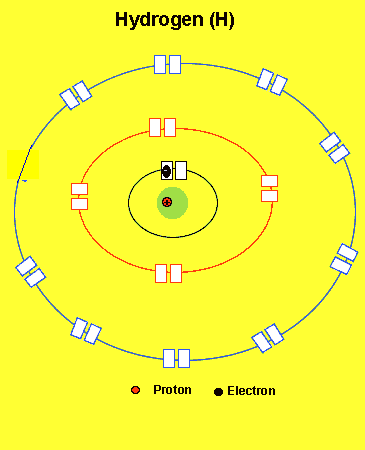The atom Filling its energy levels. Lets see how the energy levels of different atoms fill. Look at the animation below. Notice how the total number of electrons equal the number of protons in the nucleus. A neutral atom has equal protons and electrons. Note, also, how the lowest energy levels fill first and once full the atom becomes very stable.We write the electronic configuration of the elements in simple terms as shown below. H =1 (one electron in the first energy level) He =2 (two electrons in the first energy level) Li = 2,1(two electrons in the first energy level and one in the second level) Be = 2,2(two electrons in the first energy level and two in the second level) B =2,3(two electrons in the first energy level and threein the second level) C =2,4(two electrons in the first energy level and four in the second level) N =2,5(two electrons in the first energy level and five in the second level) O =2,6(two electrons in the first energy level and six in the second level) F =2,7(two electrons in the first energy level and seven in the second level) Ne =2,8(two electrons in the first energy level and eight in the second level) Na = 2,8,1(two electrons in the first level, eight in the second and one in the third ) Continue